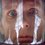# Playing with Integrals: Limits and Integrals 3

Here comes the last part of the Limits and Integrals series, previous ones can be found here: Part 1 and Part 2. This time we will discuss even more challenging limits, so get ready and have fun!

The next problem was found in "A Note on Evaluating Limits Using Riemann Sums" by Sudhir K. Goel and Dennis M. Rodriguez.

Problem 7. Evaluate $\lim_{n\to\infty}\left(\frac{1}{n}\ln(n!)-\ln n\right)$

Solution. After mastering several similar problems there should be no dificulty in applying our technique to this one. $\lim_{n\to\infty}\left(\frac{1}{n}\ln(n!)-\ln n\right)=\lim_{n\to\infty}\frac{1}{n}(\ln(n!)-n\ln n)=$ $=\lim_{n\to\infty}\frac{1}{n}\left(\sum^n_{i=1}\ln\left(\frac{i}{n}\right)\right)=\int^1_0\ln x\,dx.$

Even slightest knowledge of integrals suggest that something went wrong. Actually everything is just fine, but the intgeral we need to evaluate is an improper one. Now let's proceed to finish it $\int^1_0\ln x\,dx=\left. (xlnx-x)\right|^1_0=-1+\lim_{x\to 0}(x\ln x)=-1.$

Now let's move onto the next example.

Problem 8. Calculate $\lim_{n\to\infty}\left(\sin\frac{1}{\sqrt{n^2+1}}+\sin\frac{1}{\sqrt{n^2+4}}+...+\sin\frac{1}{\sqrt{10n^2}}\right)$

Solution. This one will be a tough task, but after eight solved examples nothing is imposible.

$\lim_{n\to\infty}\left(\sum^{3n}_{i=1}\sin\frac{1}{\sqrt{n^2+i^2}}\right)=\lim_{n\to\infty}\left(\sum^{3n}_{i=1}\sin\frac{1}{n\sqrt{1+\frac{i^2}{n^2}}}\right).$

Now we need to get rid of the $\sin$ function. Many of you proably know that near the $0$ function $sin x$ behaves just like $x$. This fact is proven by computing Taylor Series of $sin x$ at the point $x=0$.

$\lim_{n\to\infty}\left(\sum^{3n}_{i=1}\sin\frac{1}{n\sqrt{1+\frac{i^2}{n^2}}}\right)=\lim_{n\to\infty}\left(\sum^{3n}_{i=1}\frac{1}{n\sqrt{1+\frac{i^2}{n^2}}}\right)+$ $+O\left(\lim_{n\to\infty}\sum^{3n}_{i=1}\left(\frac{1}{n\sqrt{1+\frac{i^2}{n^2}}}\right)^3\right)=\int^3_0\frac{1}{\sqrt{x^2+1}}\,dx=$ $=\boxed{\ln(3+\sqrt{10})}.$

The last problem in this series of posts we'll be following one.

Problem 9. Find the limit $\lim_{n\to\infty}\frac{\sqrt[n]{n!}}{n}$Note by Nicolae Sapoval
7 years ago

This discussion board is a place to discuss our Daily Challenges and the math and science related to those challenges. Explanations are more than just a solution — they should explain the steps and thinking strategies that you used to obtain the solution. Comments should further the discussion of math and science.

When posting on Brilliant:

• Use the emojis to react to an explanation, whether you're congratulating a job well done , or just really confused .
• Ask specific questions about the challenge or the steps in somebody's explanation. Well-posed questions can add a lot to the discussion, but posting "I don't understand!" doesn't help anyone.
• Try to contribute something new to the discussion, whether it is an extension, generalization or other idea related to the challenge.

MarkdownAppears as
*italics* or _italics_ italics
**bold** or __bold__ bold
- bulleted- list
• bulleted
• list
1. numbered2. list
1. numbered
2. list
Note: you must add a full line of space before and after lists for them to show up correctly
paragraph 1paragraph 2

paragraph 1

paragraph 2

[example link](https://brilliant.org)example link
> This is a quote
This is a quote
    # I indented these lines
# 4 spaces, and now they show
# up as a code block.

print "hello world"
# I indented these lines
# 4 spaces, and now they show
# up as a code block.

print "hello world"
MathAppears as
Remember to wrap math in $$ ... $$ or $ ... $ to ensure proper formatting.
2 \times 3 $2 \times 3$
2^{34} $2^{34}$
a_{i-1} $a_{i-1}$
\frac{2}{3} $\frac{2}{3}$
\sqrt{2} $\sqrt{2}$
\sum_{i=1}^3 $\sum_{i=1}^3$
\sin \theta $\sin \theta$
\boxed{123} $\boxed{123}$

Sort by:

Well done , Nicolae :)

_Problem 9 _

Solution: $\displaystyle \lim_{n \to \infty} \frac{\sqrt[n] {n!}}{n}$

= $\displaystyle \lim_{n \to \infty} {\bigg(\frac{ {n!}}{n^n}\bigg)}^{\frac{1}{n}}$

= $\displaystyle \lim_{n \to \infty} {\bigg(\prod_{r= 1}^{n} \frac{r}{n}\bigg)}^{\frac{1}{n}}$

= $\displaystyle \lim_{n \to \infty} \text{exp}\bigg(\frac{1}{n} \sum_{r=1}^{n} \ln \bigg(\frac{r}{n}\bigg)\bigg)$

= $\displaystyle \text{exp} \bigg(\displaystyle \int_{0}^{1} \ln x \text{dx} \bigg)$

We know by using by parts that $\displaystyle \int \ln x \text{dx} = x \ln x - x$, and we also know that $\displaystyle \lim_{x \to 0} x \ln x = 0$, hence, the integral $\displaystyle \int_{0}^{1} \ln x \text{dx}$ turns out to be $-1$, hence, finally, we conclude that answer to the problem is $\boxed{e^{-1}}$

I had a question abt latex, actually, i wanted to know how do we put limits on an indefinte integral , when solving a definite integral on latex.

If you don't mind , i add problem 10 here:

Problem 10 Evaluate $\displaystyle \lim_{n \to \infty} \displaystyle \prod_{r=1}^{2n} \bigg(\frac{r+n}{n}\bigg)^{\frac{r}{n^2}}$

- 7 years ago

Nice post again Nicolae! :)

Solution to Problem 10:

The given limit is equivalent to

$\displaystyle \lim_{n\rightarrow \infty} \exp\left(\frac{1}{n}\sum_{r=1}^{2n} \frac{r}{n}\ln\left(1+\frac{r}{n}\right)\right)$

= $\displaystyle \exp\left(\int_0^2 x\ln(1+x)\,\text{d}x \right)$

The above integral can be easily evaluated using integration by parts and is equal to $(3/2)\ln2$. Hence the final answer is $2\sqrt{2}$.

- 7 years ago

Shouldn't it be $3\sqrt{3}$ ? As $\displaystyle \int_0^{2} x\ln(1+x) \mathrm d x=(3/2)\ln3$

- 7 years ago

You are right, sorry about that. :)

- 7 years ago

No problem bro :D

- 7 years ago

P.S. I hope that during the next few days I'll make/find about 4-5 problems involving this technique and will add them as problems. I also will post a note leading to all 3 posts and related problems.

- 7 years ago

Nice Job!

- 7 years ago

Really good job bud.

- 7 years ago